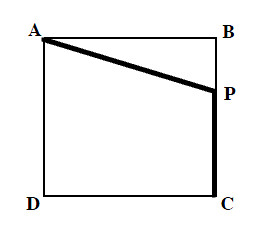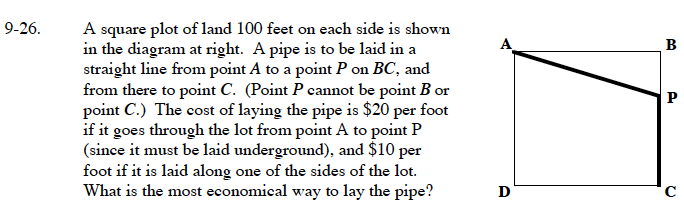### Home > CALC > Chapter 9 > Lesson 9.1.2 > Problem9-26

9-26.

A square plot of land 100 feet on each side is shown in the diagram below. A pipe is to be laid in a straight line from point A to a point P on BC, and from there to point C. (Point P cannot be point B or point C.) The cost of laying the pipe is $20 per foot if it goes through the lot from point A to point P (since it must be laid underground), and$10 per foot if it is laid along one of the sides of the lot. What is the most economical way to lay the pipe? Homework Help ✎Let x = BP.
Now write expressions for AB and CP.

The function for the cost is y = 20(AP) + 10(CP).

Once you have written the cost function in terms of x, differentiate and determine the value of x that yields a miniumum.

x ≈ 57.735 ft